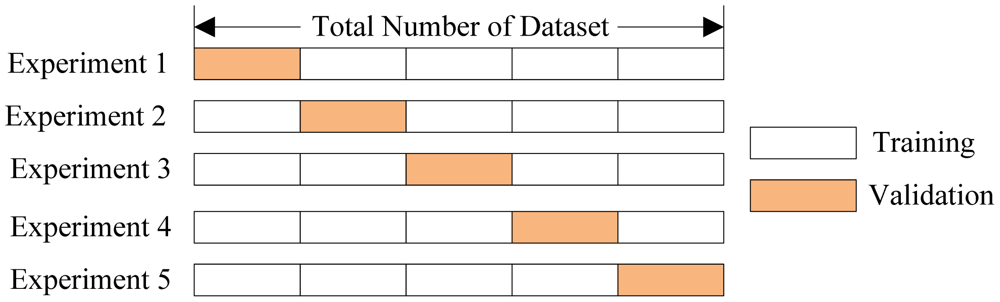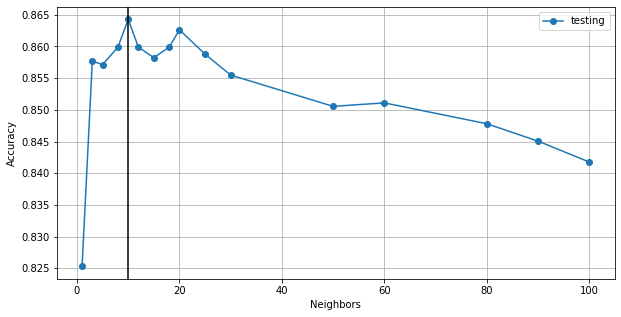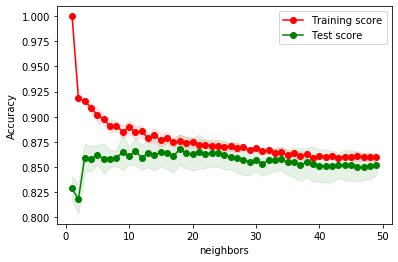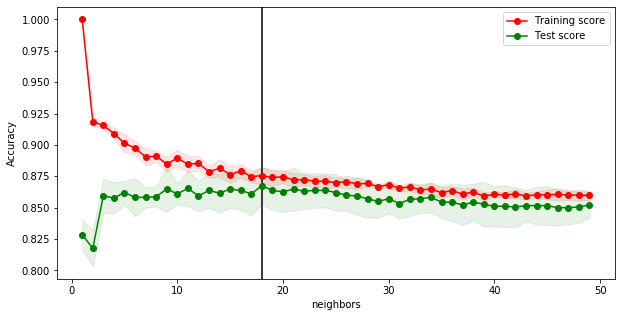# MLF - Cross-Validation and Parameter Tuning

Last updated: July 13th, 2020# Cross-validation and parameter tuning¶

In this lesson we will continue the machine learning application previously. In the process, we will introduce some machine learning core concepts and terms.## Remember the problem¶

Over the past few years, streaming services with huge catalogs have become the primary means through which most people listen to their favorite music. But at the same time, the sheer amount of music on offer can mean users might be a bit overwhelmed when trying to look for newer themes that suits their tastes.For this reason, streaming services have looked into means of categorizing music to allow for personalized recommendations. One method involves direct analysis of the raw audio information in a given song, scoring the raw data on a variety of metrics.

In this lesson we'll be examining data compiled by a research group known as The Echo Nest.

Our goal is to look through this dataset and classify songs as being either 'Hip-Hop' or 'Rock' - all without listening to a single one ourselves. In doing so, we will learn how to clean our data and do some exploratory data visualization towards the goal of feeding our data through a simple machine learning algorithm.## Get the data and our latest model¶

We will get tracks data as we left it in previous lesson, and use it to train a KNeighborsClassifier model as we did before.

In :
import numpy as np
import pandas as pd
import matplotlib.pyplot as plt

tracks = pd.read_csv('tracks_3.csv')

In :
tracks.head()

Out:
track_id acousticness danceability energy instrumentalness liveness speechiness tempo valence genre_top genre_top_code
0 3681 0.624076 0.294289 0.856591 0.891003 0.115368 0.041453 117.741 0.250994 Rock 1
1 37637 0.628078 0.361321 0.825037 0.847185 0.196486 0.039852 139.139 0.599086 Rock 1
2 41302 0.801959 0.506456 0.688173 0.888620 0.141702 0.047079 92.209 0.653078 Rock 1
3 40948 0.846783 0.227621 0.469203 0.933570 0.080574 0.029546 80.122 0.945517 Rock 1
4 837 0.405611 0.244638 0.837132 0.725711 0.095129 0.049809 142.916 0.307880 Rock 1
In :
tracks.info()

<class 'pandas.core.frame.DataFrame'>
RangeIndex: 1820 entries, 0 to 1819
Data columns (total 11 columns):
#   Column            Non-Null Count  Dtype
---  ------            --------------  -----
0   track_id          1820 non-null   int64
1   acousticness      1820 non-null   float64
2   danceability      1820 non-null   float64
3   energy            1820 non-null   float64
4   instrumentalness  1820 non-null   float64
5   liveness          1820 non-null   float64
6   speechiness       1820 non-null   float64
7   tempo             1820 non-null   float64
8   valence           1820 non-null   float64
9   genre_top         1820 non-null   object
10  genre_top_code    1820 non-null   int64
dtypes: float64(8), int64(2), object(1)
memory usage: 156.5+ KB


#### Select Features ($X$) and Labels ($y$)¶

In :
X = tracks.drop(['track_id', 'genre_top', 'genre_top_code'], axis=1)
y = tracks['genre_top_code']


#### Train and Test sets¶

In :
from sklearn.model_selection import train_test_split

X_train, X_test, y_train, y_test = train_test_split(X, y,
test_size=0.2,
random_state=10)


#### Data normalization¶

We will use the StandardScaler to standardize the features (X_train and X_test) before moving to model creation.

In :
from sklearn.preprocessing import StandardScaler

scaler = StandardScaler()

scaler.fit(X_train)

X_train = scaler.transform(X_train)
X_test = scaler.transform(X_test)


#### Build and train the model¶

In :
from sklearn.neighbors import KNeighborsClassifier

k=5
model = KNeighborsClassifier(n_neighbors=k)

In :
model.fit(X_train, y_train)

Out:
KNeighborsClassifier(algorithm='auto', leaf_size=30, metric='minkowski',
metric_params=None, n_jobs=None, n_neighbors=5, p=2,
weights='uniform')

#### Make predictions¶

In :
y_pred = model.predict(X_test)


#### Evaluate the model¶

In :
from sklearn.metrics import classification_report

model_report = classification_report(y_test, y_pred)

print("Model report: \n", model_report)

Model report:
precision    recall  f1-score   support

0       0.87      0.80      0.84       184
1       0.81      0.88      0.84       180

accuracy                           0.84       364
macro avg       0.84      0.84      0.84       364
weighted avg       0.84      0.84      0.84       364## Cross-validation to evaluate our model¶

Cross validation (CV) works by defining multiple experiments to run in our sample data. It's a little bit more resource intensive, but it'll let us have a better evaluation of our parameters and model.

CV attempts to split the data multiple ways and test the model on each of the splits.### Performing cross-validation¶

We will use what's known as K-fold CV here which first splits the data into K different, equally sized subsets. Then, it iteratively uses each subset as a test set while using the remainder of the data as train sets.

First we define the strategy to split the dataset, selecting one of the many built-in.

In this case, we will use k-fold, with k=5 "folds" (as seen in the picture).

In :
from sklearn.model_selection import KFold

kf = KFold(n_splits=5, random_state=10)

/usr/local/lib/python3.8/site-packages/sklearn/model_selection/_split.py:292: FutureWarning: Setting a random_state has no effect since shuffle is False. This will raise an error in 0.24. You should leave random_state to its default (None), or set shuffle=True.
warnings.warn(


We import the cross_val_score, that will compute the score for our estimator.

In :
from sklearn.model_selection import cross_val_score


We now need to build the estimator with those parameters that we want to evaluate.

In :
model = KNeighborsClassifier(n_neighbors=5)


We now use the entire dataset, as it'll be split internally by the cross validator.

cv=kf indicates to use the k-fold split strategy

In :
X = np.concatenate((X_train, X_test))
X.shape

Out:
(1820, 8)
In :
y = np.concatenate((y_train, y_test))
y.shape

Out:
(1820,)
In :
scores = cross_val_score(model, X, y, cv=kf)

scores

Out:
array([0.87637363, 0.85164835, 0.87087912, 0.86538462, 0.84065934])

Finally, we can then aggregate the results from each fold for a final model performance score.

In :
print("Accuracy: %0.2f (+/- %0.2f)" % (scores.mean(), scores.std() * 2))

Accuracy: 0.86 (+/- 0.03)


Important: As cross-validation tests our model with different data, the less standard deviation we get on every cross-validation score, the more robust our model will be.

### Cross-validation predictions¶

We can also generate cross-validated estimated for each input data point using cross-validation.

In :
from sklearn.model_selection import cross_val_predict

model = KNeighborsClassifier(n_neighbors=5)

y_pred = cross_val_predict(model, X, y,
cv=KFold(n_splits=5, random_state=10))

y_pred

/usr/local/lib/python3.8/site-packages/sklearn/model_selection/_split.py:292: FutureWarning: Setting a random_state has no effect since shuffle is False. This will raise an error in 0.24. You should leave random_state to its default (None), or set shuffle=True.
warnings.warn(

Out:
array([1, 0, 1, ..., 1, 0, 1])
In :
model_report = classification_report(y, y_pred)

print("Model report: \n", model_report)

Model report:
precision    recall  f1-score   support

0       0.88      0.84      0.86       910
1       0.84      0.89      0.86       910

accuracy                           0.86      1820
macro avg       0.86      0.86      0.86      1820
weighted avg       0.86      0.86      0.86      1820


In :
from sklearn.metrics import confusion_matrix

conf_matrix = confusion_matrix(y, y_pred)
conf_matrix

Out:
array([[761, 149],
[104, 806]])

### Cross-validation¶

cross_validate. is a similar function, but a bit more versatile and informative, than cross_val_score. In addition to returning the scores, it returns some others metrics that may be useful, such as trained models, etc. In addition, it allows evaluating more than just one metric.

In :
from sklearn.model_selection import cross_validate

In :
scores = cross_validate(model, X, y, cv=kf)

In :
print(scores)

{'fit_time': array([0.00172138, 0.00167274, 0.0014832 , 0.00142074, 0.00139642]), 'score_time': array([0.0516212 , 0.0200882 , 0.02018499, 0.0202024 , 0.02943635]), 'test_score': array([0.87637363, 0.85164835, 0.87087912, 0.86538462, 0.84065934])}


The next cell prints a list of all the metrics that we can use to evaluate with cross_validate

In :
import sklearn
sorted(sklearn.metrics.SCORERS.keys())

Out:
['accuracy',
'adjusted_mutual_info_score',
'adjusted_rand_score',
'average_precision',
'balanced_accuracy',
'completeness_score',
'explained_variance',
'f1',
'f1_macro',
'f1_micro',
'f1_samples',
'f1_weighted',
'fowlkes_mallows_score',
'homogeneity_score',
'jaccard',
'jaccard_macro',
'jaccard_micro',
'jaccard_samples',
'jaccard_weighted',
'max_error',
'mutual_info_score',
'neg_brier_score',
'neg_log_loss',
'neg_mean_absolute_error',
'neg_mean_gamma_deviance',
'neg_mean_poisson_deviance',
'neg_mean_squared_error',
'neg_mean_squared_log_error',
'neg_median_absolute_error',
'neg_root_mean_squared_error',
'normalized_mutual_info_score',
'precision',
'precision_macro',
'precision_micro',
'precision_samples',
'precision_weighted',
'r2',
'recall',
'recall_macro',
'recall_micro',
'recall_samples',
'recall_weighted',
'roc_auc',
'roc_auc_ovo',
'roc_auc_ovo_weighted',
'roc_auc_ovr',
'roc_auc_ovr_weighted',
'v_measure_score']## Model parameters tuning¶

On previous sections we trained a KNeighborsClassifier with 5 neighbors using the neighbors=5 parameter, but is this the best amount of neighbors? Can we boost our model by tunning this parameter?

All estimators have parameters (often called hyper-parameters in the literature) that can be tuned. The generalization power of an estimator often critically depends on a few parameters.

Quite often, it is not clear what the exact values of model parameters should be since they depend on the data at hand.

In :
def get_kneighbors_score(k):
model = KNeighborsClassifier(n_neighbors=k)
scores = cross_val_score(model, X, y, cv=5)
return "Accuracy: %0.3f (+/- %0.3f)" % (scores.mean(), scores.std() * 2)

In :
get_kneighbors_score(5)

Out:
'Accuracy: 0.862 (+/- 0.019)'
In :
get_kneighbors_score(2)

Out:
'Accuracy: 0.818 (+/- 0.029)'
In :
get_kneighbors_score(15)

Out:
'Accuracy: 0.865 (+/- 0.030)'
In :
get_kneighbors_score(40)

Out:
'Accuracy: 0.851 (+/- 0.032)'

#### Validation curve:¶

Now we could visualize the relation between the hyper-parameter 'k' and the accuracy.

In :
parameters = [1, 3, 5, 8, 10, 12, 15, 18, 20, 25, 30, 50,60,80,90,100]

def get_kneighbors_score(k):
model = KNeighborsClassifier(n_neighbors=k)
scores = cross_val_score(model, X, y, cv=4)
return scores.mean()

ACC_dev = []
for k in parameters:
scores=get_kneighbors_score(k)
ACC_dev.append(scores)

print(ACC_dev)

[0.8252747252747252, 0.8576923076923078, 0.8571428571428572, 0.8598901098901099, 0.8642857142857143, 0.8598901098901099, 0.8582417582417583, 0.8598901098901099, 0.8626373626373627, 0.8587912087912088, 0.8554945054945056, 0.8505494505494506, 0.8510989010989012, 0.8478021978021979, 0.8450549450549449, 0.8417582417582417]


Let's plot the accuracy versus the number of neighbors

In :
f, ax = plt.subplots(figsize=(10,5))
plt.plot(parameters,ACC_dev,'o-', label='testing')
plt.axvline(x=10, ymin=0, ymax=1,color='k')
plt.xlabel('Neighbors')
plt.ylabel('Accuracy')
plt.grid()
plt.legend()
plt.plot()

print( 'Best parameters:', 12, 'Accuracy:' , 0.75)

Best parameters: 12 Accuracy: 0.75The Validation curve (or training curve) shows the validation and training score of an estimator for varying numbers of neighbors. To complete develop the curve we need to use cross_validate

Now we could visualize the relation between the hyper-parameter 'k' and the accuracy of training and testing using cross_validate

In :
from sklearn.model_selection import cross_validate
knn_train_scores_mean = []
knn_train_scores_std = []
knn_test_scores_mean = []
knn_test_scores_std = []

k = np.arange(1,50,1)

for neighbors in k:
clf = KNeighborsClassifier(n_neighbors=neighbors)
knn_scores = cross_validate(clf, X, y, cv=5, return_train_score=True, n_jobs = -1)

knn_train_scores_mean.append(knn_scores['train_score'].mean())
knn_train_scores_std.append(knn_scores['train_score'].std())

knn_test_scores_mean.append(knn_scores['test_score'].mean())
knn_test_scores_std.append(knn_scores['test_score'].std())

knn_train_scores_mean = np.array(knn_train_scores_mean)
knn_train_scores_std = np.array(knn_train_scores_std)
knn_test_scores_mean = np.array(knn_test_scores_mean)
knn_test_scores_std = np.array(knn_test_scores_std)


Plot the accuracy versus the number of neighbors

In :
plt.fill_between(k, knn_train_scores_mean - knn_train_scores_std,
knn_train_scores_mean + knn_train_scores_std, alpha=0.1,
color="r")
plt.fill_between(k, knn_test_scores_mean - knn_test_scores_std,
knn_test_scores_mean + knn_test_scores_std, alpha=0.1, color="g")

plt.plot(k, knn_train_scores_mean, 'o-', color="r",
label="Training score")
plt.plot(k, knn_test_scores_mean, 'o-', color="g",
label="Test score")

plt.legend()
plt.ylabel('Accuracy')
plt.xlabel('neighbors')
plt.show()Which is the best k parameter?

In :
f, ax = plt.subplots(figsize=(10,5))
plt.axvline(x=18, ymin=0, ymax=1,color='k')
plt.fill_between(k, knn_train_scores_mean - knn_train_scores_std,
knn_train_scores_mean + knn_train_scores_std, alpha=0.1,
color="r")
plt.fill_between(k, knn_test_scores_mean - knn_test_scores_std,
knn_test_scores_mean + knn_test_scores_std, alpha=0.1, color="g")

plt.plot(k, knn_train_scores_mean, 'o-', color="r",
label="Training score")
plt.plot(k, knn_test_scores_mean, 'o-', color="g",
label="Test score")

plt.legend()
plt.ylabel('Accuracy')
plt.xlabel('neighbors')
plt.show()Scikit-learn also provides tools to automatically find the best parameter combinations (via cross-validation), we will go through this methodologies in the course Modeling selection and evaluation